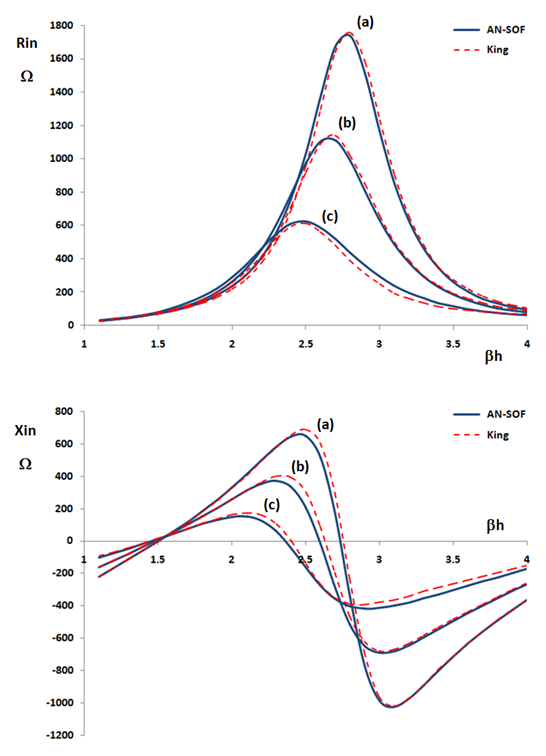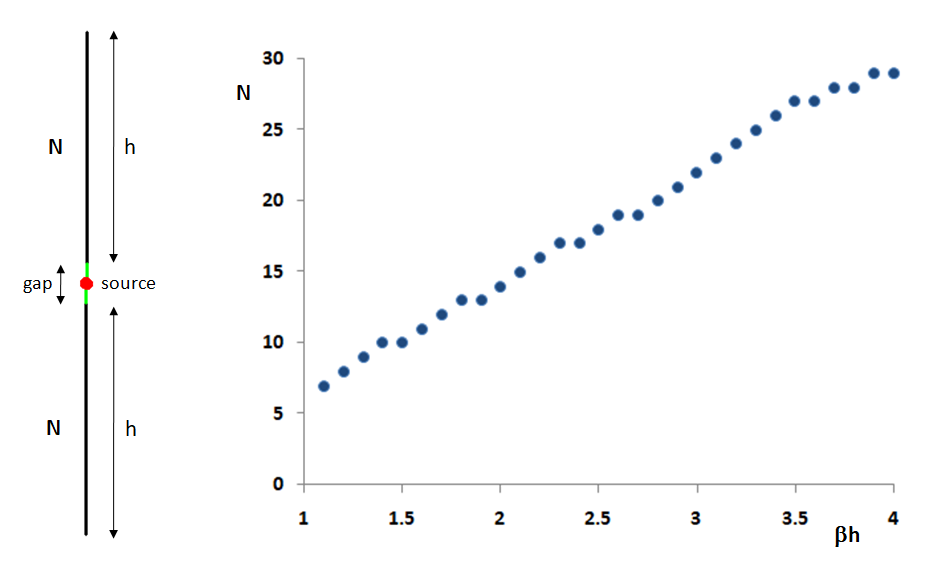How can we help?

# Impedance of Cylindrical Antennas

Since there is a lot of theoretical data available for the input impedance of a linear antenna, we will compare the simulation results with those obtained theoretically.

Among the theoretical results, we have chosen the King-Middleton’s because from our point of view it is the most elaborate analytical solution. It is based on an expansion of the current distribution along a linear antenna in terms of sinusoidal functions and integrals of those functions, which is used in an iterative method to approach the solution of an integral equation. However, King-Middleton’s solution should not be taken as completely “correct” as it neglects edge effects at the antenna feed point, so the gap where the source is connected is considered to be infinitesimal. Besides, the King-Middleton’s solution is just a second order approximation. Even today it is in doubt if this solution really converges when more iterations are performed. Needless to say, it is impressive that an analytical second-order iteration of the solution of an integral equation could be obtained in King’s days.

Beyond these observations, the King-Middleton second-order solution can be taken as a good reference to validate a numerical method.

Fig. 1 shows the input impedance of a center-fed cylindrical antenna as a function of its normalized half-length, ßh (in radians), ß being the wavenumber. For a wavelength λ = 1 m, the antenna diameter is (a) 3/32″ (radius/λ = 0.001191), (b) 1/4″ (radius/λ = 0.003175), (c) 3/4″ (radius/λ = 0.009525). The solid lines are AN-SOF simulation results while the dashed lines are King’s results. Refer to Table 30.12 of “The Theory of Linear Antennas” by Ronold W. P. King, Harvard Univ. Press, 1956.Fig. 1: Rin (Xin) is the real (imaginary) part of the input impedance of a center-fed cylindrical antenna. ß = 2π/λ is the wavenumber, h is the dipole half-length. For a wavelength λ = 1 m, the antenna diameter is (a) 3/32″, (b) 1/4″, (c) 3/4″.

Since King’s results neglect the finite gap effect at the source position, the following gap sizes have been used in AN-SOF in order to better approximate the King’s curves: (a) 0.02λ, (b) 0.04λ, (c) 0.1λ. In the AN-SOF model the antenna does not have any gap, in fact, since the continuity of the electric current must be guaranteed along the antenna. To fill in the gap, a short wire having the same length as the gap is used. The source feeding the antenna is at the center of this short wire.

Fig. 2 shows the AN-SOF model. The number of segments was increased as ßh increases in order to maintain a practically constant segment length of 0.02λ. This segment length is more than enough to obtain a convergent value of the input impedance, as the convergence analysis will show. Fig. 2 also shows the number of segments N used in each arm of the antenna as a function of ßh, which is practically a linear function of course.Fig. 2: Model of a cylindrical antenna in AN-SOF and the number of segments used in each antenna arm as a function of the normalized arm length, ßh.

As can be seen, an excellent agreement is achieved between the King-Middleton second-order approximation and the results obtained from AN-SOF.